Objective :
To study the vibration of an acoustic string under tension.
Primary language: Fortran.

Principle :

We considered a string of length L and a known linear density ρ(x), which is fixed at both ends and subjected to a tension of intensity σ. The following differential equation allows us to describe the vibration of the string: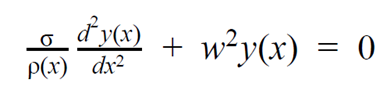The Dirichlet boundary conditions are: y(0) = y(L) = 0 and y(x) represents the position of a material point of the string on the y-axis.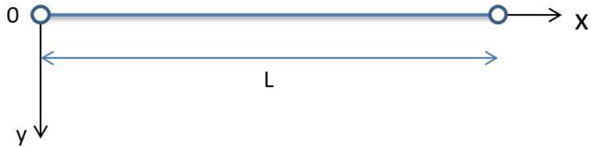To solve this problem, we must look for solutions of this differential equation, which are the proper pulsations ωk and the eigenmodes yk(x).

The numerical approach that we use is the finite difference method which first discretizes the field of study in N regular intervals with a step of h = L and then it approaches the N derivatives of y(x) with linear combinations. The second derivative of y(x) can therefore be rewritten: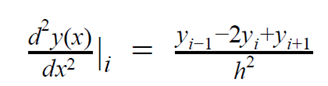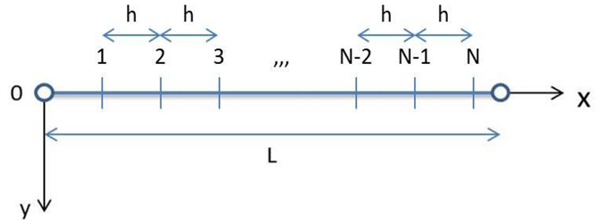With these two equations and the Dirichlet boundary conditions, we can write our system of N equations in matrix form: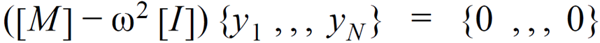where [M] is a positive definite symmetric matrix and [I] is the identity matrix.

Programmed Methods :
• Matrix Generator of [M]
• Descent Method
• Ascent Method
• LU Decomposition Method
• Cholesky Decomposition Method
• Inverse Power Method - Cholesky Decomposition
• SOR Method
• Inverse Power Method - SOR
• Power Iteration Method
• Deflation Method
Fortran Implementation :